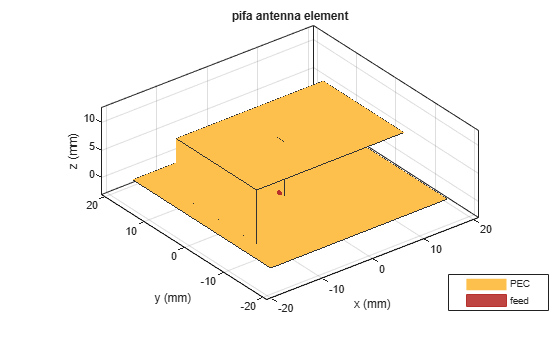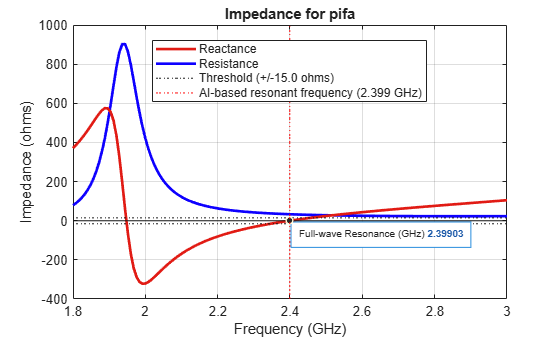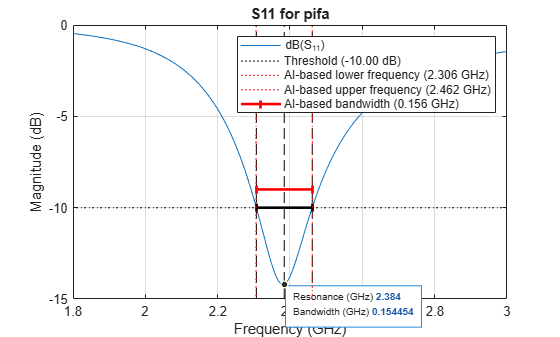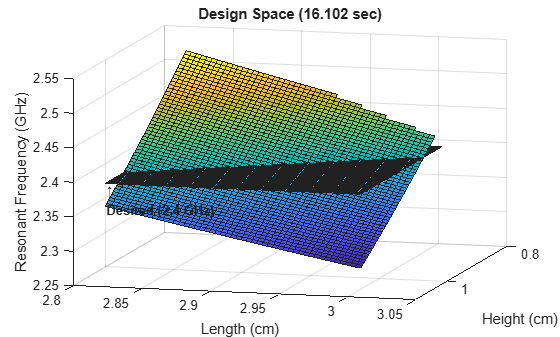# Artificial Intelligence (AI) for Rapid Analysis and Design of Microstrip Patch Antenna

This example shows how to use artificial intelligence (AI) to rapidly analyze and design antennas from the Antenna Toolbox™ catalog.

Learn how the `AIAntenna` object can be used to take advantage of the speed of AI-based analysis without the need for prior machine learning experience. Follow this example to:

1. Create an antenna for AI-based analysis using `AIAntenna.`

2. Calculate the resonant frequency for a specific antenna design.

3. Rapidly explore the antenna across the design space.

### Create Antenna for AI-Based Analysis

Using `design` with `ForAI=true`, create an `AIAntenna` for AI-based analysis of a microstrip patch antenna designed to resonate around 2.4 GHz.

```antType = patchMicrostrip; fd = 2.4e9; pmAI = design(antType,fd,ForAI=true);```

Calculate the resonant frequency of the prototype antenna using AI-based analysis by calling `resonantFrequency` on the `AIAntenna` object.

`resonantFrequencyAI0 = resonantFrequency(pmAI)`
```resonantFrequencyAI0 = 2.3965e+09 ```

Note that the calculated resonant frequency is approximately equal to the design frequency of 2.4 GHz.

### Calculate Resonant Frequency of Specific Antenna Design

To achieve a higher bandwidth , increase the width to be 1.45 times the length.

First, query the `AIAntenna` object. The tunable parameters of a microstrip patch antenna for AI-based analysis are length, width, and height.

`pmAI`
```pmAI = AIAntenna with properties: Antenna Info AntennaType: 'patchMicrostrip' InitialDesignFrequency: 2.4000e+09 Tunable Parameters Length: 0.0600 Width: 0.0781 Height: 0.0012 Use 'showReadOnlyProperties(pmAI)' to show read-only properties ```

To view the nontunable properties by calling `showReadOnlyProperties`.

`showReadOnlyProperties(pmAI)`
``` SubstrateName: 'Air' SubstrateEpsilonR: 1 SubstrateLossTangent: 0 SubstrateThickness: 0.0012 GroundPlaneLength: 0.1249 GroundPlaneWidth: 0.1249 PatchCenterOffset: [0 0] FeedOffset: [0.0126 0] ConductorName: 'PEC' ConductorConductivity: Inf ConductorThickness: 0 Tilt: 0 TiltAxis: [1 0 0] LoadImpedance: [] LoadFrequency: [] LoadLocation: 'feed' ```

Define the desired design specification.

`W = pmAI.Length*1.45`
```W = 0.0869 ```

Before you can modify your prototype to meet the desired design, use `tunableRanges` to verify that your desired value is within the tunable ranges supported for AI-based analysis of your antenna. In this case, the desired width of 0.0869 m is in the tunable range of 0.0664 m to 0.0898 m.

`tRanges = tunableRanges(pmAI)`
```tRanges=1×3 table Length Width Height min max min max min max ____________________ ___________________ ______________________ resonantFrequency 0.050965 0.068952 0.06636 0.089782 0.0010618 0.0014365 ```
`assert((W>=tRanges.Width.min) && (W<=tRanges.Width.max))`

Specify the antenna width as the desired value.

`pmAI.Width = W;`

Use the `show` function to visualize the design.

`show(pmAI)`#### Calculate Resonant Frequency Using AI-Based Analysis

Use AI-based analysis to calculate the resonant frequency for your antenna. The AI-based calculation shows the resonant frequency of the antenna to be 2.3923 GHz.

```tic resonantFrequencyAI = resonantFrequency(pmAI)```
```resonantFrequencyAI = 2.3934e+09 ```
`timeAI = toc;`

The AI-based calculation indicates that increasing the length of the antenna decreases the resonant frequency.

#### Calculate Resonant Frequency Using Full-wave EM Solver

To perform the full-wave EM solver simulation on your antenna, export the design from the `AIAntenna` object by calling the `exportAntenna` function.

`pmAnt = exportAntenna(pmAI);`

Run the full-wave EM solver on the exported antenna design. Get the resonant frequency by calculating the frequency at which the reactance of the antenna is zero. To perform this calculation, use the helper function `getZeroCrossing` provided in this example.

```fSweep = linspace(0.5,1.5,101)*fd; tic z = impedance(pmAnt,fSweep); timeFull = toc; resonantFrequencyFull = getZeroCrossing(imag(z),fSweep);```

#### Compare AI-Based Results with Full-wave EM Solver Results

Plot the results of the full-wave EM solver against the results of the AI-based analysis. The resonant frequency from the AI-based analysis is nearly indistinguishable from the full-wave EM solver results. Although the relative error is less than 1%, the AI-based calculations are significantly faster than the the full-wave EM solver calculation time.

```impedance(pmAnt,fSweep) xlim([fSweep(1) fSweep(end)]*1e-9) fResGHz = [resonantFrequencyAI resonantFrequencyFull]*1e-9; hold on xline(fResGHz(1),"--k") xline(fResGHz(2),"-k") legend(["Resistance" "Reactance" ... sprintf("AI-based resonant frequency (%1.3f GHz)",fResGHz(1)), ... sprintf("Full-wave resonant frequency (%1.3f GHz)",fResGHz(2))], ... Location="northwest")```In the figure, the resonant frequency from AI-based analysis is nearly indistinguishable from full-wave EM solver results.

Now compare the results in a table.

```absErr = abs(resonantFrequencyAI - resonantFrequencyFull); relativeErrorPercent = 100*absErr/resonantFrequencyFull; tErr = table(resonantFrequencyAI,resonantFrequencyFull,relativeErrorPercent)```
```tErr=1×3 table resonantFrequencyAI resonantFrequencyFull relativeErrorPercent ___________________ _____________________ ____________________ 2.3934e+09 2.3958e+09 0.098212 ```
`tTime = table(timeAI,timeFull)`
```tTime=1×2 table timeAI timeFull ________ ________ 0.051881 186.02 ```

### Rapidly Explore and Characterize Antenna Across Design Space

Optimize your antenna design by reducing the height by 10%, but maintain the same the resonant frequency and width-to-length ratio. To find the desired length, use AI-based analysis to rapidly explore this design space.

First, define the parameters for tuning the height and length incrementally to characterize the space by using `defaultTunableParameters` to track the default values. To maintain the resonant frequency, specify the tuning range of the length to be a narrow range, because the length is the resonant dimension  and the resonant frequency of your antenna is already relatively close to the desired frequency.

```n = 50; hScaler = linspace(0.86,1.14,n); lScaler = linspace(0.968,1.032,n); H0 = pmAI.defaultTunableParameters.Height; L0 = pmAI.defaultTunableParameters.Length;```

Keeping the width-to-length ratio locked at 1.45, explore the design space by calculating the resonant frequency with respect to the antenna's height and length.

```fResAI = zeros(n); tic for hIdx = 1:n for lIdx = 1:n pmAI.Height = H0*hScaler(hIdx); pmAI.Length = L0*lScaler(lIdx); pmAI.Width = pmAI.Length*1.45; fResAI(lIdx,hIdx) = resonantFrequency(pmAI); end end timeAI1 = toc;```

Visualize the AI-based calculations of the resonant frequency across the design space.

`fig = figure`
```fig = Figure (2) with properties: Number: 2 Name: '' Color: [0.9400 0.9400 0.9400] Position: [348 376 583 437] Units: 'pixels' Use GET to show all properties ```
```surf(hScaler*H0*100,lScaler*L0*100,fResAI*1e-9) view(105,15) xlabel("Height (cm)") ylabel("Length (cm)") zlabel("Resonant Frequency (GHz)") title(sprintf("Design Space (%1.3f sec)",timeAI1))```

Plot where the resonant frequency intersects with the desired value of 2.4 GHz.

```hold on surf(hScaler*H0*100,lScaler*L0*100,ones(n)*fd*1e-9, ... FaceAlpha=0) text(H0*hScaler(end)*100,L0*lScaler(1)*100,fd*1e-9, ... strcat("\uparrow",sprintf("\nDesired (%1.1f GHz)\n",fd*1e-9)), ... VerticalAlignment="top", ... FontWeight="bold")```Notice that you were able to calculate 50 * 50 = 2500 points in the design space within a couple of seconds. This would not have been practical using full-wave EM analysis due to the substantially longer computation time.

Calculate the desired size-reduced height.

`H1 = H0*0.9;`

Find the desired length based on this desired height. Inspect the design space to find the point where the resonant frequency is 2.4 GHz and the height is 0.0011 m.

`L1 = 0.0601641`
```L1 = 0.0602 ```

Calculate the resonant frequency at the desired length and height using AI-based analysis, and visualize the point which the desired length and height was inspected.

```pmAI.Height = H1; pmAI.Length = L1; pmAI.Width = pmAI.Length*1.45; F1 = resonantFrequency(pmAI); s = fig.CurrentAxes.Children(2); s.DataTipTemplate.DataTipRows(1).Label = "Height"; s.DataTipTemplate.DataTipRows(2).Label = "Length"; s.DataTipTemplate.DataTipRows(3).Label = "Resonant Frequency"; dt = datatip(s,H1*100,L1*100,F1*1e-9);``````lengthFinal = pmAI.Length; heightFinal = pmAI.Height; widthFinal = pmAI.Width; resonantFrequencyFinalAI = F1; tFinal = table(lengthFinal,heightFinal,widthFinal,resonantFrequencyFinalAI)```
```tFinal=1×4 table lengthFinal heightFinal widthFinal resonantFrequencyFinalAI ___________ ___________ __________ ________________________ 0.060164 0.0011242 0.087238 2.4012e+09 ```

### Analyze AI-Based Antenna Design

Finally, run the full-wave EM solver on your final antenna.

```pmAntFinal = exportAntenna(pmAI); z = impedance(pmAntFinal,fSweep); resonantFrequencyFinalFull = getZeroCrossing(imag(z),fSweep);```

Verify that the AI-based design of your antenna was accurate by comparing it against the full-wave EM solver results.

```absErrFinal = abs(resonantFrequencyFinalAI - resonantFrequencyFinalFull); relativeErrorFinal = absErrFinal/resonantFrequencyFinalFull; tErrFinal = table(resonantFrequencyFinalAI,resonantFrequencyFinalFull, ... relativeErrorFinal)```
```tErrFinal=1×3 table resonantFrequencyFinalAI resonantFrequencyFinalFull relativeErrorFinal ________________________ __________________________ __________________ 2.4012e+09 2.3951e+09 0.0025567 ```

### Summary of AI-Based Analysis

AI-based analysis allows for the characterization of antennas with:

• Faster calculation time

• High accuracy

This enables workflows that were not previously practical. As demonstrated in this example, you can explore a design space with high resolution within a couple of seconds.

With `AIAntenna`, you can take advantage of AI-based analysis without prior machine learning experience.

### Reference

 Jackson, David. "Chapter 7: Microstrip Antennas," in Antenna Engineering Handbook, edited by John Volakis, 4th ed., New York: McGraw-Hill Co., 2007.